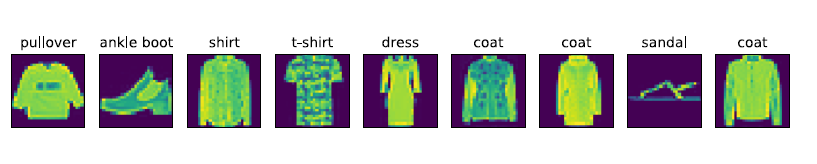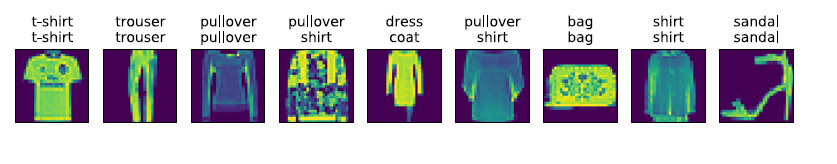# 图像分类数据集（Fashion-MNIST）

## 1、获取数据集

### 1.1 获取数据集

In : %matplotlib inline
import gluonbook as gb
from mxnet.gluon import data as gdata
import sys
import time


In : mnist_train = gdata.vision.FashionMNIST(train=True)
mnist_test = gdata.vision.FashionMNIST(train=False)


In : len(mnist_train), len(mnist_test)
Out: (60000, 10000)


In : feature, label = mnist_train


In : feature.shape, feature.dtype
Out: ((28, 28, 1), numpy.uint8)


In : label, type(label), label.dtype
Out: (2, numpy.int32, dtype('int32'))


Fashion-MNIST 是⼀个10 类服饰分类数据集。Fashion-MNIST 中⼀共包括了10 个类别，分别为：t-shirt（T 恤）、trouser（裤⼦）、pullover（套衫）、dress（连⾐裙）、coat（外套）、sandal（凉鞋）、shirt（衬衫）、sneaker（运动鞋）、bag（包）和ankle boot（短靴）。以下函数可以将数值标签转成相应的⽂本标签。

In : # 本函数已保存在gluonbook 包中⽅便以后使⽤。
def get_fashion_mnist_labels(labels):
text_labels = ['t-shirt', 'trouser', 'pullover', 'dress', 'coat',
'sandal', 'shirt', 'sneaker', 'bag', 'ankle boot']
return [text_labels[int(i)] for i in labels]


In : # 本函数已保存在gluonbook 包中⽅便以后使⽤。
def show_fashion_mnist(images, labels):
gb.use_svg_display()
# 这⾥的_ 表⽰我们忽略（不使⽤）的变量。
_, figs = gb.plt.subplots(1, len(images), figsize=(12, 12))
for f, img, lbl in zip(figs, images, labels):
f.imshow(img.reshape((28, 28)).asnumpy())
f.set_title(lbl)
f.axes.get_xaxis().set_visible(False)
f.axes.get_yaxis().set_visible(False)


In : X, y = mnist_train[0:9]
show_fashion_mnist(X, get_fashion_mnist_labels(y))### 1.2 读取小批量

batch_size 的小批量数据。这⾥的批量⼤小batch_size 是⼀个超参数。

In : batch_size = 256
transformer = gdata.vision.transforms.ToTensor()
if sys.platform.startswith('win'):
num_workers = 0        # 0 表⽰不⽤额外的进程来加速读取数据。
else:
num_workers = 4
batch_size, shuffle=True,
num_workers=num_workers)
batch_size, shuffle=False,
num_workers=num_workers)


In : start = time.time()
for X, y in train_iter:
continue
'%.2f sec' % (time.time() - start)
Out: '1.09 sec'


## 2、Softmax 回归的从零开始实现

In : %matplotlib inline
import gluonbook as gb


### 2.1 获取和读取数据

In : batch_size = 256


### 2.2 初始化模型参数

In : num_inputs = 784
num_outputs = 10
W = nd.random.normal(scale=0.01, shape=(num_inputs, num_outputs))
b = nd.zeros(num_outputs)


In : W.attach_grad()


### 2.3 实现Softmax 运算

In : X = nd.array([[1, 2, 3], [4, 5, 6]])
X.sum(axis=0, keepdims=True), X.sum(axis=1, keepdims=True)

Out: (
[[5. 7. 9.]]
<NDArray 1x3 @cpu(0)>,
[[ 6.]
[15.]]
<NDArray 2x1 @cpu(0)>)


Softmax 运算的输出矩阵中的任意⼀⾏元素代表了⼀个样本在各个输出类别上的预测概率。

In : def softmax(X):
X_exp = X.exp()
partition = X_exp.sum(axis=1, keepdims=True)
return X_exp / partition # 这⾥应⽤了⼴播机制。


In : X = nd.random.normal(shape=(2, 5))
X_prob = softmax(X)
X_prob, X_prob.sum(axis=1)

Out: (
[[0.21324193 0.33961776 0.1239742 0.27106097 0.05210521]
[0.11462264 0.3461234 0.19401033 0.29583326 0.04941036]]
<NDArray 2x5 @cpu(0)>,
[1.0000001 1. ]
<NDArray 2 @cpu(0)>)


### 2.4 定义模型

In : def net(X):
return softmax(nd.dot(X.reshape((-1, num_inputs)), W) + b)


### 2.5 定义损失函数

“Softmax 回归”⼀节数学表述中标签类别离散值从1 开始逐⼀递增不同，在代码中，标签类别的

In : y_hat = nd.array([[0.1, 0.3, 0.6], [0.3, 0.2, 0.5]])
y = nd.array([0, 2])
nd.pick(y_hat, y)

Out:
[0.1 0.5]
<NDArray 2 @cpu(0)>


In : def cross_entropy(y_hat, y):
return - nd.pick(y_hat, y).log()


### 2.6 计算分类准确率

⼤元素的索引，且返回结果与变量y 形状相同。我们在“数据操作”⼀节介绍过，相等条件判断

In : # 本函数已保存在gluonbook 包中⽅便以后使⽤。
def accuracy(y_hat, y):
return (y_hat.argmax(axis=1) == y.astype('float32')).mean().asscalar()


⼀致。因此，这两个样本上的分类准确率为0.5。

In : accuracy(y_hat, y)
Out: 0.5


In : # 本函数已保存在gluonbook 包中⽅便以后使⽤。该函数将被逐步改进：它的完整实现将在“图像增⼴”⼀节中描述。
def evaluate_accuracy(data_iter, net):
acc = 0
for X, y in data_iter:
acc += accuracy(net(X), y)
return acc / len(data_iter)


0.1。

In : evaluate_accuracy(test_iter, net)
Out: 0.0947265625


### 2.7 训练模型

In : num_epochs, lr = 5, 0.1
# 本函数已保存在gluonbook 包中⽅便以后使⽤。
def train_ch3(net, train_iter, test_iter, loss, num_epochs, batch_size,
params=None, lr=None, trainer=None):
for epoch in range(num_epochs):
train_l_sum = 0
train_acc_sum = 0
for X, y in train_iter:
y_hat = net(X)
l = loss(y_hat, y)
l.backward()
if trainer is None:
gb.sgd(params, lr, batch_size)
else:
trainer.step(batch_size) # 下⼀节将⽤到。
train_l_sum += l.mean().asscalar()
train_acc_sum += accuracy(y_hat, y)
test_acc = evaluate_accuracy(test_iter, net)
print('epoch %d, loss %.4f, train acc %.3f, test acc %.3f'
% (epoch + 1, train_l_sum / len(train_iter),
train_acc_sum / len(train_iter), test_acc))
train_ch3(net, train_iter, test_iter, cross_entropy, num_epochs,
batch_size, [W, b], lr)

Out : epoch 1, loss 0.7867, train acc 0.749, test acc 0.798
epoch 2, loss 0.5744, train acc 0.810, test acc 0.825
epoch 3, loss 0.5295, train acc 0.822, test acc 0.831
epoch 4, loss 0.5049, train acc 0.831, test acc 0.830
epoch 5, loss 0.4903, train acc 0.834, test acc 0.838


### 2.8 预测

In : for X, y in test_iter:
break
true_labels = gb.get_fashion_mnist_labels(y.asnumpy())
pred_labels = gb.get_fashion_mnist_labels(net(X).argmax(axis=1).asnumpy())
titles = [true + '\n' + pred for true, pred in zip(true_labels, pred_labels)]
gb.show_fashion_mnist(X[0:9], titles[0:9])©️2019 CSDN 皮肤主题: 大白 设计师: CSDN官方博客